## Goodman's Formula

A two-coloring of a Complete Graphofnodes which contains exactly the number of Monochromatic Forced Triangles and no more (i.e., a minimum of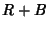whereand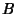are the number of red and blue Triangles) is called an Extremal Graph. Goodman (1959) showed that for an extremal graph,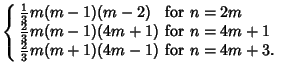Schwenk (1972) rewrote the equation in the form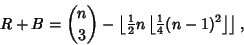whereis a Binomial Coefficient and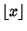is the Floor Function.

Goodman, A. W. On Sets of Acquaintances and Strangers at Any Party.'' Amer. Math. Monthly 66, 778-783, 1959.
Schwenk, A. J. Acquaintance Party Problem.'' Amer. Math. Monthly 79, 1113-1117, 1972.